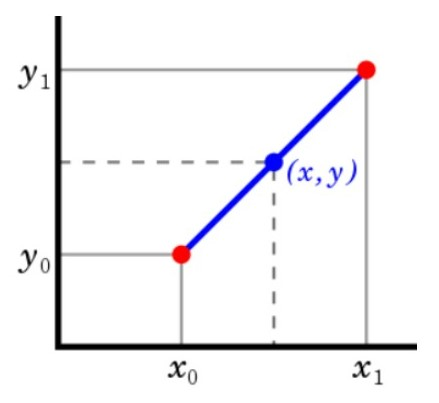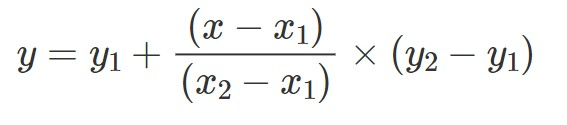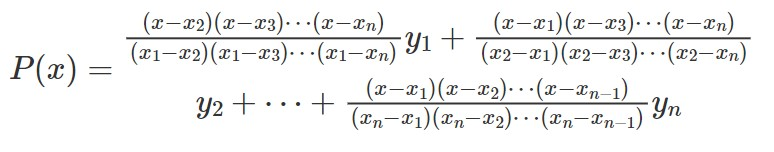# Interpolation in Python – How to interpolate missing data, formula and approaches

Interpolation can be used to impute missing data. Let’s see the formula and how to implement in Python.

But, you need to be careful with this technique and try to really understand whether or not this is a valid choice for your data. Often, interpolation is applicable when the data is in a sequence or a series.

You should also know there are multiple interpolation methods available, the default is a linear method.

## When to use interpolation for imputing missing data?

You can use interpolation when there is an order or a sequence and you want to estimate a missing value in the sequence. For example: Let’s say there are various classes of tickets in train travel, like, first class, second class, and so on. You would naturally expect the ticket price of the higher class to be more expensive than the lower class.

In that case, if the ticket price of an intermediate class is missing, you can use interpolation to estimate the missing value.

When not to use interpolation?

In case, there was no association between the order of the classes and the ticket fares, that is, if it was not necessary that the first class is more expensive than the second class, then, it might not be appropriate to use interpolation.

Let’s see this with an example.

import numpy as np
import pandas as pd

# class and ticket prices.
fare = {'first_class':100,
'second_class':np.nan,
'third_class':60,
'open_class':20}


Convert it to a pandas series object to make interpolation convenient.

# store as pandas series
ser = pd.Series(fare)
ser

first_class     100.0
second_class      NaN
third_class      60.0
open_class       20.0
dtype: float64


Now you can use ser.interpolate() to predict the missing value. By default, ser.interpolate() will do a linear interpolation.

## Important caveat before you apply interpolation

Linear interpolation will take the index (0,1,2..) as the X and the column you want to interpolate as Y and do the interpolation. So, you need to make sure the X is sorted in your data to make this work.In the above equation, when ‘x’ is known, you can compute the value of ‘y’, using the the following formula for linear interpolation.Interpolation is also possible on a multi-dimensional space as well and is given by La-grange’s interpolation polynomial.## Implement linear interpolation

ser.interpolate(method='linear')

first_class     100.0
second_class     80.0
third_class      60.0
open_class       20.0
dtype: float64


linear interpolation may be more suitable if you assume the relationship between x (index) and y (value) to be linear. If not, you might want to try spline and cubicspline interpolation as well.

Spline interpolation

To use spline interpolation you need to make sure the index is reset to start from 0,1,2.. etc. So do a reset_index first, then do interpolate.

# order = 2
ser.reset_index(drop=True).interpolate(method='spline', order=2)

0    100.000000
1     86.666667
2     60.000000
3     20.000000
dtype: float64


Cubic spline

# cubic spline
ser.reset_index(drop=True).interpolate(method='cubicspline')

0    100.000000
1     86.666667
2     60.000000
3     20.000000
dtype: float64


## Related Topics

Build Your First Machine Learning Project – ML Lessons Series

## Similar Articles

### Logistic Regression – A Complete Tutorial With Examples in RCourse Preview

## Machine Learning A-Z™: Hands-On Python & R In Data Science

### Free Sample Videos:#### Machine Learning A-Z™: Hands-On Python & R In Data Science#### Machine Learning A-Z™: Hands-On Python & R In Data Science#### Machine Learning A-Z™: Hands-On Python & R In Data Science#### Machine Learning A-Z™: Hands-On Python & R In Data Science